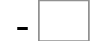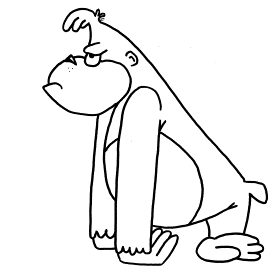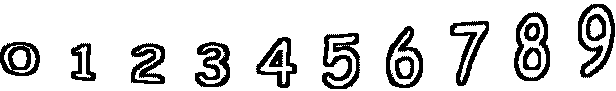6= 6   5= 53= 3   1= 1   0= 0   4= 0 8= 7   1= 0   6= 0   9= 1